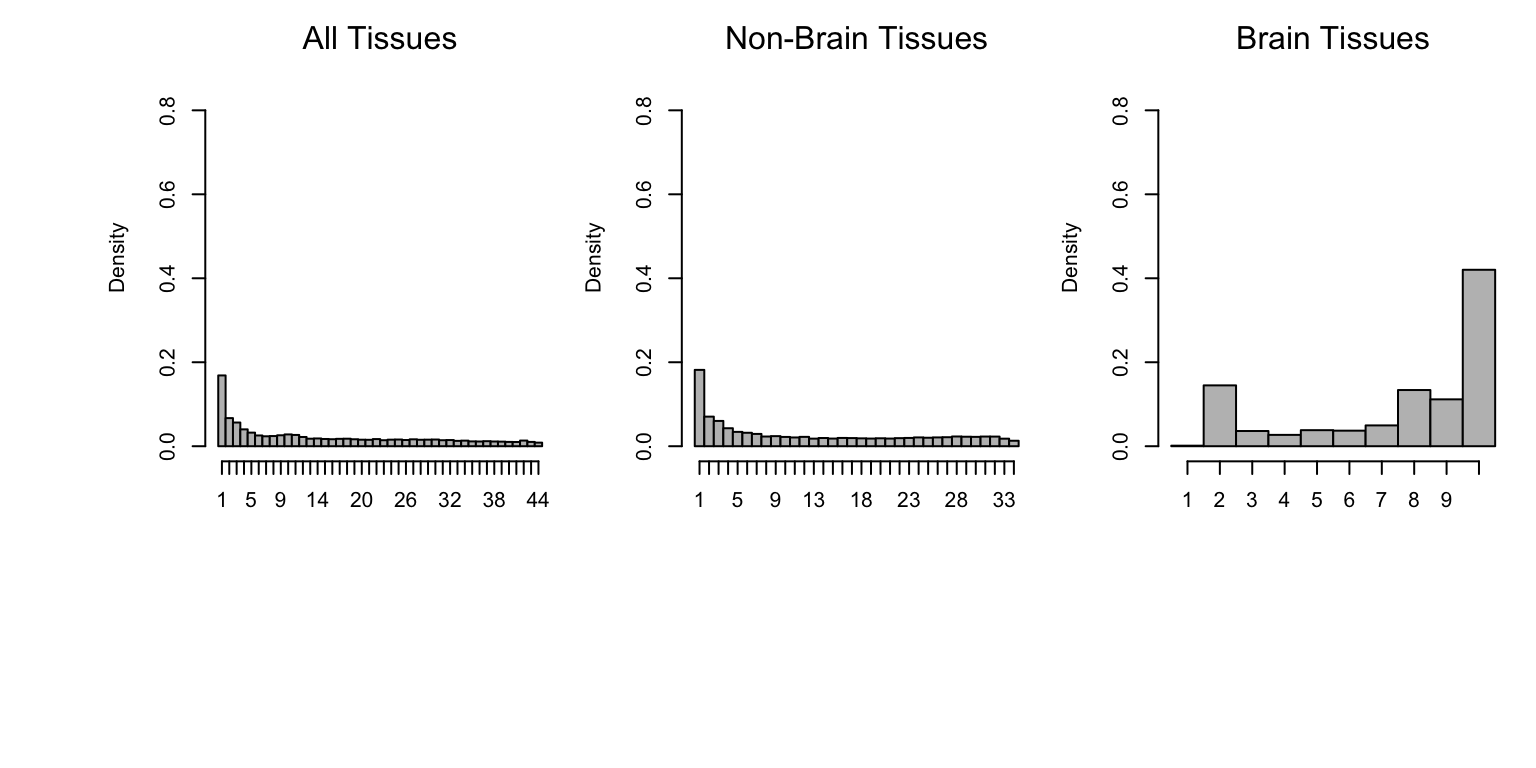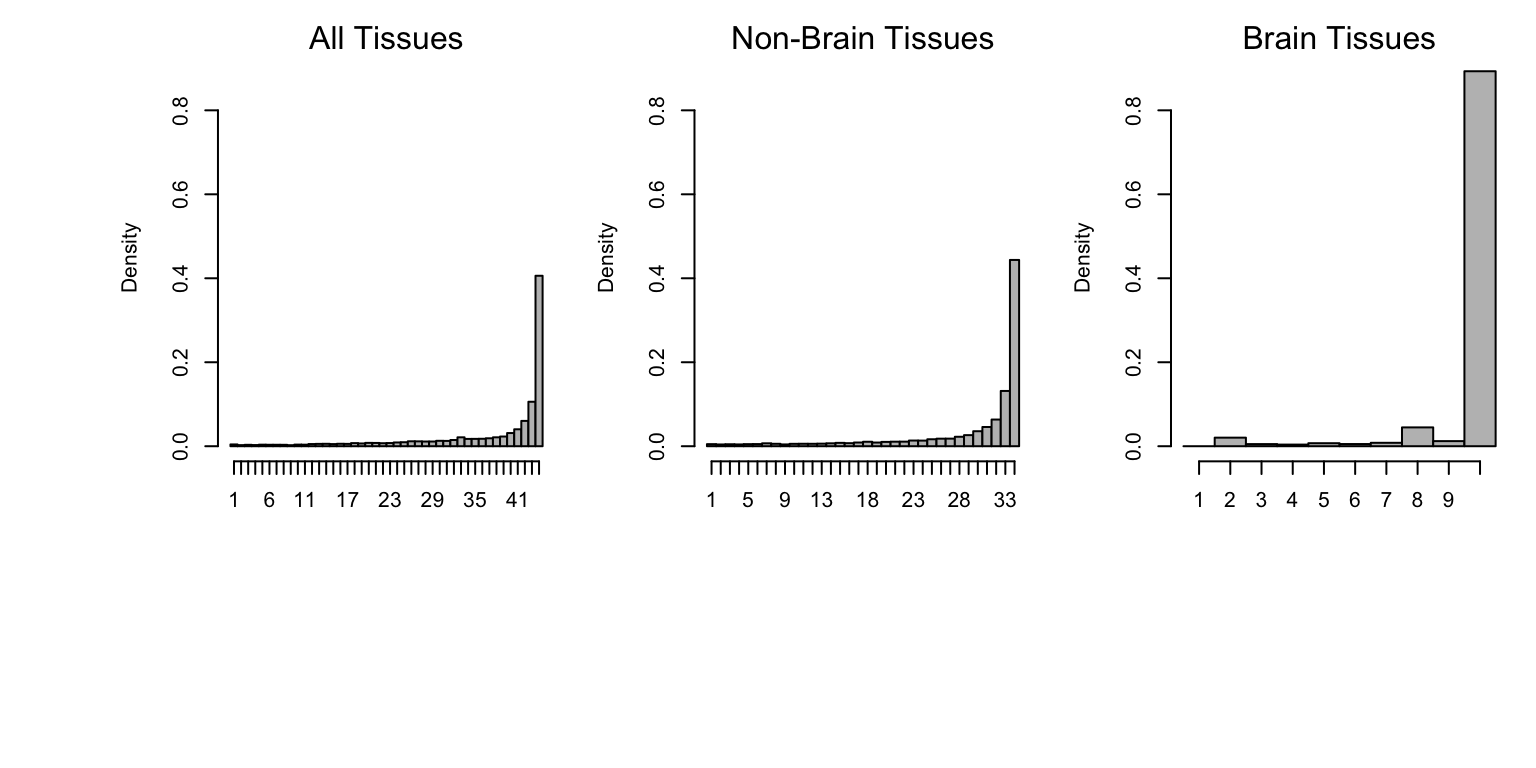Last updated: 2018-06-06

• R Markdown file: up-to-date

Great! Since the R Markdown file has been committed to the Git repository, you know the exact version of the code that produced these results.

• Environment: empty

Great job! The global environment was empty. Objects defined in the global environment can affect the analysis in your R Markdown file in unknown ways. For reproduciblity it’s best to always run the code in an empty environment.

• Seed: set.seed(1)

The command set.seed(1) was run prior to running the code in the R Markdown file. Setting a seed ensures that any results that rely on randomness, e.g. subsampling or permutations, are reproducible.

• Session information: recorded

Great job! Recording the operating system, R version, and package versions is critical for reproducibility.

• Repository version: 5d5a403

Great! You are using Git for version control. Tracking code development and connecting the code version to the results is critical for reproducibility. The version displayed above was the version of the Git repository at the time these results were generated.

Note that you need to be careful to ensure that all relevant files for the analysis have been committed to Git prior to generating the results (you can use wflow_publish or wflow_git_commit). workflowr only checks the R Markdown file, but you know if there are other scripts or data files that it depends on. Below is the status of the Git repository when the results were generated:

Ignored files:
Ignored:    .sos/
Ignored:    data/.sos/
Ignored:    output/MatrixEQTLSumStats.Portable.Z.coved.K3.P3.lite.single.expanded.V1.loglik.rds
Ignored:    workflows/.ipynb_checkpoints/
Ignored:    workflows/.sos/

Untracked files:
Untracked:  fastqtl_to_mash_output/
Untracked:  gtex6_workflow_output/


Note that any generated files, e.g. HTML, png, CSS, etc., are not included in this status report because it is ok for generated content to have uncommitted changes.
Expand here to see past versions:
File Version Author Date Message
Rmd 5d5a403 Peter Carbonetto 2018-06-06 wflow_publish(c(“ExpressionAnalysis.Rmd”, “fastqtl2mash.Rmd”,
html 7ca37fb Peter Carbonetto 2018-06-06 Build site.
Rmd 0226fe3 Peter Carbonetto 2018-06-06 wflow_publish(“SharingHist.Rmd”)
Rmd ddd3bd3 Peter Carbonetto 2018-06-06 wflow_publish(“SharingHist.Rmd”)
html 0c91e3f Peter Carbonetto 2018-06-06 I have a first complete revision of the SharingHist analysis without
Rmd 0f4a56a Peter Carbonetto 2018-06-06 wflow_publish(“SharingHist.Rmd”)
Rmd d199d66 Peter Carbonetto 2018-06-06 wflow_publish(“Tspecific.Rmd”)

Here we develop another summary of sharing of eQTLs across tissues by showing the estimated distribution of sharing; specifically, the distribution of the number of tissues shared by sign and by magnitude. This complements this summary and this one.

Compare the plots below to the plots in Figure 5 of the paper.

## Set up environment

First, we load some functions defined for the mash analyses.

source("../code/normfuncs.R")

This is the threshold used to determine whether a gene has an eQTL in a given tissue.

thresh <- 0.05

## Load data and mash results

Load some GTEx summary statistics, as well as some of the results generated from the mash analysis of the GTEx data.

out     <- readRDS("../data/MatrixEQTLSumStats.Portable.Z.rds")
maxbeta <- out$test.b maxz <- out$test.z
"lite.single.expanded.V1.posterior.rds",sep = "."))
lfsr           <- out$lfsr pm.mash <- out$posterior.means
standard.error <- maxbeta/maxz
pm.mash.beta   <- pm.mash * standard.error

# Histograms of sharing by magnitude

Sharing by magnitude means that two eQTLs have similar effect size (within a factor of 2).

The histograms below summarize sharing by magnitude across all tissues (left), tissues other than brain (middle), and brain tissues only (right).

sigmat <- (lfsr <= thresh)
nsig   <- rowSums(sigmat)
par(mar = c(4,4,2,1))
par(oma = c(8,4,0,0) + 0.1)
par(mfrow = c(1,3))
hist((het.func(het.norm(effectsize=pm.mash.beta[nsig>0,]),threshold=0.5)),
main="",xlab="",breaks=0.5:44.5,col="grey",freq=FALSE,ylim=c(0,0.9),
xaxt="n")
axis(1,at = seq(1, 44, by=1),labels = c(1:44))
mtext("All Tissues")

sigmat <- (lfsr[,-c(7:16)] <= thresh)
nsig   <- rowSums(sigmat)
hist((het.func(het.norm(effectsize=pm.mash.beta[nsig>0,-c(7:16)]),
threshold=0.5)),main="",xlab="",breaks=0.5:34.5,col="grey",
freq=FALSE,ylim=c(0,0.9),xaxt="n")
axis(1,at = seq(1, 34, by=1),labels = c(1:34))
mtext("Non-Brain Tissues")

sigmat     <- (lfsr[,c(7:16)]<=thresh)
nsig       <-  rowSums(sigmat)
brain.norm <- het.norm(effectsize=pm.mash.beta[nsig>0,c(7:16)])
hist(het.func(brain.norm,threshold=0.5),main="",xlab="",breaks=0.5:10.5,
col="grey",freq=FALSE,xaxt="n",ylim=c(0,0.9))
axis(1, at=seq(1, 10, by=1), labels=c(1:10))
mtext("Brain Tissues")Expand here to see past versions of hindex-plot-magnitude-1.png:
Version Author Date
0c91e3f Peter Carbonetto 2018-06-06

Observe that the distribution of the number of tissues in which an eQTL is shared by magnitude has a mode at 1. This is a subset of eQTLs that have much stronger effect in one tissue than in any other (“tissue-specific”).

## Histogram of sharing by magnitude

Sharing by sign means that the eQTLs have the same sign of effect.

The histograms below summarize sharing by sign across all tissues (left), tissues other than brain (middle), and brain tissues only (right).

sign.func <- function (normeffectsize)
apply(normeffectsize,1,function(x)(sum(x>0)))
sigmat <- (lfsr<=thresh)
nsig   <- rowSums(sigmat)
par(mar = c(4,4.5,2,1))
par(oma = c(8,4,0,0) + 0.1)
par(mfrow = c(1,3))
hist(sign.func(het.norm(effectsize=pm.mash.beta[nsig>0,])),main="",xlab="",
breaks=0.5:44.5,col="grey",freq=FALSE,xaxt="n",ylim=c(0,0.9))
axis(1, at=seq(1, 44, by=1), labels=c(1:44))
mtext("All Tissues")

sigmat <- (lfsr[,-c(7:16)] <= thresh)
nsig   <- rowSums(sigmat)
hist(sign.func(het.norm(effectsize = pm.mash.beta[nsig>0,-c(7:16)])),
main="",xlab="",breaks=0.5:34.5,col="grey",freq=FALSE,ylim=c(0,0.9),
xaxt="n")
axis(1, at=seq(1, 34, by=1), labels=c(1:34))
mtext("Non-Brain Tissues")

sigmat     <- (lfsr[,c(7:16)]<=thresh)
nsig       <-  rowSums(sigmat)
brain.norm <- het.norm(effectsize=pm.mash.beta[nsig>0,c(7:16)])
hist(sign.func(brain.norm),main="",xlab="",breaks=0.5:10.5,col="grey",
freq=FALSE,xaxt="n",ylim=c(0,0.9))
axis(1, at=seq(1, 10, by=1), labels=c(1:10))
mtext("Brain Tissues")Expand here to see past versions of hindex-plot-sign-1.png:
Version Author Date
0c91e3f Peter Carbonetto 2018-06-06

Similar to other summaries of the mash analysis, we see that eQTLs exhibit a high level of sharing across tissues.

## Session information

sessionInfo()
# R version 3.4.3 (2017-11-30)
# Platform: x86_64-apple-darwin15.6.0 (64-bit)
# Running under: macOS High Sierra 10.13.4
#
# Matrix products: default
# BLAS: /Library/Frameworks/R.framework/Versions/3.4/Resources/lib/libRblas.0.dylib
# LAPACK: /Library/Frameworks/R.framework/Versions/3.4/Resources/lib/libRlapack.dylib
#
# locale:
#  en_US.UTF-8/en_US.UTF-8/en_US.UTF-8/C/en_US.UTF-8/en_US.UTF-8
#
# attached base packages:
#  stats     graphics  grDevices utils     datasets  methods   base
#
# loaded via a namespace (and not attached):
#   workflowr_1.0.1.9000 Rcpp_0.12.16         digest_0.6.15
#   rprojroot_1.3-2      R.methodsS3_1.7.1    backports_1.1.2
#   git2r_0.21.0         magrittr_1.5         evaluate_0.10.1
#  stringi_1.1.7        whisker_0.3-2        R.oo_1.21.0
#  R.utils_2.6.0        rmarkdown_1.9        tools_3.4.3
#  stringr_1.3.0        yaml_2.1.18          compiler_3.4.3
#  htmltools_0.3.6      knitr_1.20

This reproducible R Markdown analysis was created with workflowr 1.0.1.9000